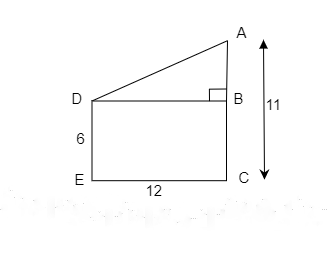# Two poles of heights 6 m and 11 m stand on a plane ground. If the distance between their feet is 12 m, find the distance between their tops.

Given:

Two poles of heights 6 m and 11 m stand on a plain ground.

The distance between their feet is 12 m.

To do:

We have to find the distance between their tops.

Solution:

Let $DE$ and $AC$ be the poles of heights $6\ m$ and $11\ m$ on the ground.The distance between their feet $EC= 12\ m$

This implies,

$DB=12\ m$

In right-angled triangle $ABD$,

By Pythagoras theorem,

$AB^2 + BD^2 = AD^2$

$5^2 + 12^2 = AD^2$   ($AB=AC-BC=11-6=5\ m$)

$AD^2 = 144 + 25$

$AD^2= 169$

$AD = \sqrt{169}=13$

Therefore, the distance between their tops is $13\ m$.

Updated on: 10-Oct-2022

29 Views